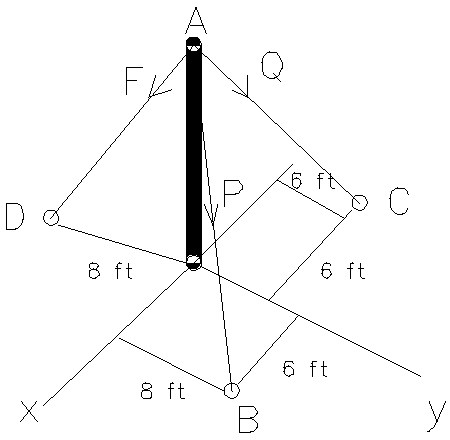# The vertical post is secured by three cables. The cables are pre-tensioned so that the resultant...

## Question:

The vertical post is secured by three cables. The cables are pre-tensioned so that the resultant of the cable forces {eq}F {/eq}, {eq}Q {/eq} and {eq}P {/eq} is directed along {eq}z {/eq}-axis. If {eq}F=120 \, lb {/eq}, find {eq}P {/eq} and {eq}Q {/eq}.## Force Balancing

When a number of forces are acting on a body, then the forces are said to be balanced if and only if the vector summation of all the forces are considered to be zero. In other words, if the net force on a body is zero, then it is said that the forces on the body are balanced.

Become a Study.com member to unlock this answer! Create your account

Given data:

• The force in the cable AD is: {eq}F = 120\;{\rm{lb}} {/eq}.
• The position vector of point B is: {eq}{\vec r_B} = \left( {6\hat i +...What is Net Force? - Definition, Magnitude & Equations

from

Chapter 7 / Lesson 7
523K

In this lesson, we will define net force, and explore the magnitude of a net force, the relevant equations and examples that will help make the concept of net force clearer.